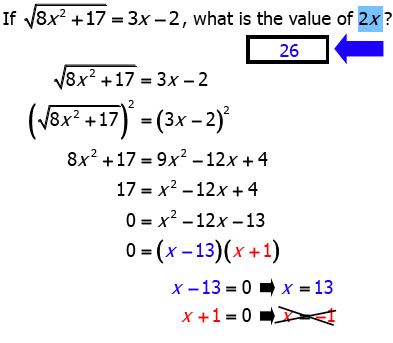It is currently 18 Jun 2019, 16:40GMAT Club Daily Prep

Thank you for using the timer - this advanced tool can estimate your performance and suggest more practice questions. We have subscribed you to Daily Prep Questions via email.

Customized
for You

we will pick new questions that match your level based on your Timer History

Track

every week, we’ll send you an estimated GMAT score based on your performance

Practice
Pays

we will pick new questions that match your level based on your Timer History

Not interested in getting valuable practice questions and articles delivered to your email? No problem, unsubscribe here.GRE Math Challenge #17 - what is the value of 2x?Question banks Downloads My Bookmarks Reviews Important topics
Author Message
TAGS:
DirectorJoined: 16 May 2014
Posts: 595
GRE 1: Q165 V161Followers: 97

Kudos [?]: 475 , given: 64

GRE Math Challenge #17 - what is the value of 2x? [#permalink]
Expert's post00:00

Question Stats:88% (01:12) correct11% (04:11) wrongbased on 9 sessions
If $$\sqrt{8x^2 +17} = 3x-2$$, what is the value of 2x? _________________________
_________________

My GRE Resources
Free GRE resources | GRE Prep Club Quant Tests
If you find this post helpful, please press the kudos button to let me know !DirectorJoined: 16 May 2014
Posts: 595
GRE 1: Q165 V161Followers: 97

Kudos [?]: 475 , given: 64

Re: GRE Math Challenge #17 [#permalink]
Expert's post
[Reveal] Spoiler: Solution_________________

My GRE Resources
Free GRE resources | GRE Prep Club Quant Tests
If you find this post helpful, please press the kudos button to let me know !InternJoined: 10 May 2015
Posts: 1
Followers: 0

Kudos [?]: 0 , given: 0

Re: GRE Math Challenge #17 [#permalink]
Why can't be the value of 'x' be -1 or -ve ?
Sorry but i'm new to GRE.
GRE InstructorJoined: 10 Apr 2015
Posts: 1981
Followers: 60

Kudos [?]: 1803 , given: 9

Re: GRE Math Challenge #17 [#permalink]
Expert's post
soumya1989 wrote:
If $$\sqrt{8x^2 +17} = 3x-2$$, what is the value of 2x? _________________________

First square both sides of the equation to get: 8x² + 17 = (3x - 2)²
Expand and simplify right side: 8x² + 17 = 9x² - 12x + 4
Rearrange to get: 0 = x² - 12x - 13
Factor to get: 0 = (x - 13)(x + 1)
So, x = 13 or x = -1

IMPORTANT: For square root equations we need to always CHECK for EXTRANEOUS roots by plugging them into the original equation.

x = 13
√[8(13²) + 17] = 3(13) - 2
Simplify to get: √[some big POSITIVE number] = 37
The important thing here is that we are finding the square root of a POSITIVE value, AND the result is also POSITIVE. PERFECT!!
As such, x = 13 is a valid solution

x = -1
√[(8)((-1)²) + 17] = 3(-1) - 2
STOP
When we evaluate the RIGHT side, we get: √[(8)((-1)²) + 17] = -5
The square root of a value cannot equal -5
So, the solution x = -1 is not valid

This means x = 13 is the only valid solution.
Since the question asks for the value of 2x, the correct answer is
[Reveal] Spoiler:
(2)(13) = 26

Cheers,
Brent
_________________

Brent Hanneson – Creator of greenlighttestprep.comInternJoined: 02 Nov 2015
Posts: 6
Followers: 0

Kudos [?]: 1 , given: 0

Re: GRE Math Challenge #17 - what is the value of 2x? [#permalink]
I would have checked x=-1 first since its easier to calculate.
DirectorJoined: 03 Sep 2017
Posts: 520
Followers: 1

Kudos [?]: 356 , given: 66

Re: GRE Math Challenge #17 [#permalink]
GreenlightTestPrep wrote:
soumya1989 wrote:
If $$\sqrt{8x^2 +17} = 3x-2$$, what is the value of 2x? _________________________

x = -1
√[(8)((-1)²) + 17] = 3(-1) - 2
STOP
When we evaluate the RIGHT side, we get: √[(8)((-1)²) + 17] = -5
The square root of a value cannot equal -5
So, the solution x = -1 is not valid

Probably, it's me that I am tired but I cannot understand how sqrt((8)((-1)^2)+17)) could be negative. -1^2 = 1 so we get sqrt(25). Am I wrong?Re: GRE Math Challenge #17   [#permalink] 07 Sep 2017, 07:13
Display posts from previous: Sort by

GRE Math Challenge #17 - what is the value of 2x?Question banks Downloads My Bookmarks Reviews Important topicsPowered by phpBB © phpBB Group Kindly note that the GRE® test is a registered trademark of the Educational Testing Service®, and this site has neither been reviewed nor endorsed by ETS®.